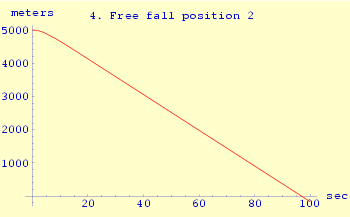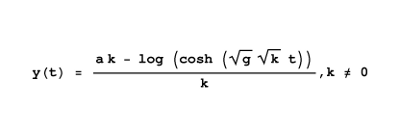Home | Mathematics | * Calculus | * Old Calculus Primer | * Old Calculus Primer 02. A Moving Experience 03. Derivation 04. Integration 05. Volume 1 06. Volume 2 07. Differential Equations 08. Terminal Velocity 09. Nature's Math 10. ToolsShare This Page
Calculus Primer 8: Terminal Velocity
P. Lutus Message Page

(double-click any word to see its definition)

Building on the result from the previous page, we will now produce a result for a falling body that accommodates air resistance. Here are the modified differential equation terms:

(1)  p''(t) = -g + p'(t)2 k
(2)  p'(0) = 0
(3)  p(0) = a

Terms 2 and 3 are identical to their previous form, but notice term 1. This term accounts for a property of air resistance — it increases as the square of velocity. It says that the acceleration experienced by our falling body is proportional to -g, a gravitational acceleration constant, plus an increasingly important air resistance term, one that eventually cancels the gravitational acceleration. As before, variable a represents the initial altitude from which the free-fall commences.The variable k is an air resistance scaling factor that encapsulates some empirical knowledge about the physical properties of falling bodies — their ratio of volume to surface area, their surface roughness and so forth. It is known that a typical skydiver achieves a terminal velocity of about 193 kilometers per hour, and we will use the meters/second equivalent of this: about 53 m/s. This requires our (empirically derived) resistance factor to be:

(4)  k = .0034

When I first wrote the above differential equation terms, because of their complexity I suspected there would be no closed-form solution (a solution expressible as a function rather than one or more numerical results). This is true of many equations of similar complexity, but as it turns out, there is a closed-form solution for this particular case:

 (5)Let's use this equation to produce some example free-fall results. You will find some graphs on this page that show various properties of the equation (and, to some degree, reality). In Graph 1, we see that, as one would expect, at the beginning of the fall when the velocity is close to zero, the acceleration is 9.8 m/s/s, e.g. simple gravitation. But as the velocity increases, air resistance builds up, and after 15 or 20 seconds there is no further significant acceleration.

Ask yourself — does "zero acceleration" mean "zero velocity"? Not in this case. Look at Graph 2, and remember that velocity is the integral of acceleration, e.g. velocity is the sum of all past accelerations, back to time zero. The terminal velocity, shown at the right, represents the sum of the accelerations for the times before air resistance balances gravitation. The graph shows that, after this balance is achieved, velocity becomes a constant.

Again, ask yourself — does a constant velocity mean a constant position? Not unless the velocity is zero. Examine Graphs 3 and 4, which show the same information using different time scales. In the same way that velocity is the sum of all past accelerations, position is the sum of all past velocities. Because velocity becomes a nonzero constant term after about 20 seconds, position changes linearly in time after that until the end of the fall.

Graph 3 expands the scale to show the first 20 seconds of the fall. It shows the expected ballistic trajectory at the beginning of the fall, before air resistance begins to balance gravitation. Graph 4 shows the entire fall and reveals that the terminal velocity is relatively constant. Had we wanted to be very precise about this result, we might have added a term to the original differential equation to take into account the change in air density at different altitudes, but most likely this modification would have sacrificed the ability to solve the equation in closed form. This change in air density has the effect of slightly slowing the descent rate as one enters the lower atmosphere.

Remember about this set of graphs that any of the derivatives, if adequately defined, can normally be used to acquire all the others, using either derivation or integration, with one limitation — when you take a derivative, you throw away a constant term from the original function. If you then decide to take an integral using that derivative, you must somehow restore that constant. In some cases this may not be important, but it is essential to remember this requirement. Expressed formally (but not too formally):

 (6)This says that one may integrate the derivative of a function f(x) to reacquire the original function (a restatement of the Fundamental Theorem of Calculus), but a discarded constant term C must be accounted for.

The business of defining and solving differential equations, of both the closed-form and numerical varieties, occupies much of the attention of applied mathematicians in many scientific and technical fields. As just one example, it is only a slight exaggeration to say that physics consists of a large set of differential equations.

 Home | Mathematics | * Calculus | * Old Calculus Primer | * Old Calculus Primer 02. A Moving Experience 03. Derivation 04. Integration 05. Volume 1 06. Volume 2 07. Differential Equations 08. Terminal Velocity 09. Nature's Math 10. ToolsShare This Page